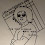### Algebra problem: airplane's speed in still air

Someone sent me this algebra problem:
An airplane flew for 6 hours with a tail wind of 60km/hr. The return flight against the same wind took 8 hours. Find the speed of the boat in still water.

Initially, this sounds like a trick problem, because you can't know the speed of the boat when all the information given is about an airplane!

But, let's assume they meant to ask the speed of the AIRPLANE in still air.

This problem has to do with constant speed. Constant speed ALWAYS involves TIME & DISTANCE.

And here we have two situations with two different speeds: First the airplane flies over there, with the wind helping. Then it flies back, and the wind is contrary of course.

It helps to organize our information in a table, once again. We'll need time, distance, and speed. And remember, speed = distance/time, or distance = speed * time. This time, time is known for both situations. Distance is not, but it is the same distance that way and back (call it d). Traveling there, the speed will be the sum of the airplane's speed (v) plus the wind speed (60). Traveling back, the wind is contrary so its speed is subtracted from the airplane's speed.

` |  Time  | Distance  | Speed------------------------------------------There |   6    |    d      | v + 60           -----------------------------------------Back |   8    |    d      | v − 60           `

I'll copy the same table here, but instead of using d for distance, I'll calculate it using distance = speed × time.

` |  Time  | Distance  | Speed------------------------------------------There |   6    |  6(v+60)  | v + 60           -----------------------------------------Back |   8    |  8(v-60)  | v − 60      `

Now, one just needs to find a way to make an equation... it will be a bit different in different word problems, but this time we get it from the fact that the distance over there is the same as the distance back:

6(v + 60) = 8(v − 60)

6v + 360 = 8v − 480

840 = 2v

v = 420

The speed of the airplane in still air is 420 km/h.

Check: For checking, we will calculate the distance it traveled. Traveling 6 hours with the speed (420 + 60) km/h or 480 km/h means the distance was 2,880 km. Traveling back took 8 hours, so the speed must have been 2,880 / 8 = 360 km/h, which is (420 − 60) km/h. So, the story "matches".Great info, I glad to see this blog, such an informative article, it really helps the student keep doing this nice work, Thanks for share thisVery nicely described solution, and always good to put in the check!

The original question is really puzzling. "Boat in still water..." Wonder what went on in the author's head. After all, no boat travels at 420km/h.Good stuff. Like to see it when math teachers KEEP IT SIMPLE. One day I'll have a blog post that shows how to show these kinds of problems with base ten manipulatives...

Maybe a little editing could be done to your post to make it say "plane" instead of "boat"...obviously two problems got edited together because the boat and current problems are exactly the same as airplane and wind problems. I always start with a guy in a canoe because the numbers are smaller and even little kids can do them once they understand the concepts...keep up the good work.Anonymous said…
very clear, neat and lovely explanation...good work!NOELIt is really a good info! I would like to visit such a unique team. All the best.Waweru said…
Assuming the airplane was hit by the wind form angle of 30 degrees what will be effect on the speedWaweru said…
What will be the best formula..Maria Miller said…
I do not know how to solve it if there's an additional wind from 30 degrees.